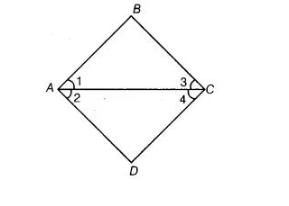`
Question:

In the adjoining figure, we have ∠1 = ∠2 and ∠2= ∠3. Show that ∠1 = ∠3.Solution:

Given, ∠1 =∠2 …(i)

and ∠2 = ∠3  …(ii)

According to Euclid’s axiom, things which are equal to the same thing are equal to one another.

From Eqs. (i) and (ii),

∠1 = ∠3